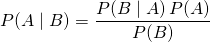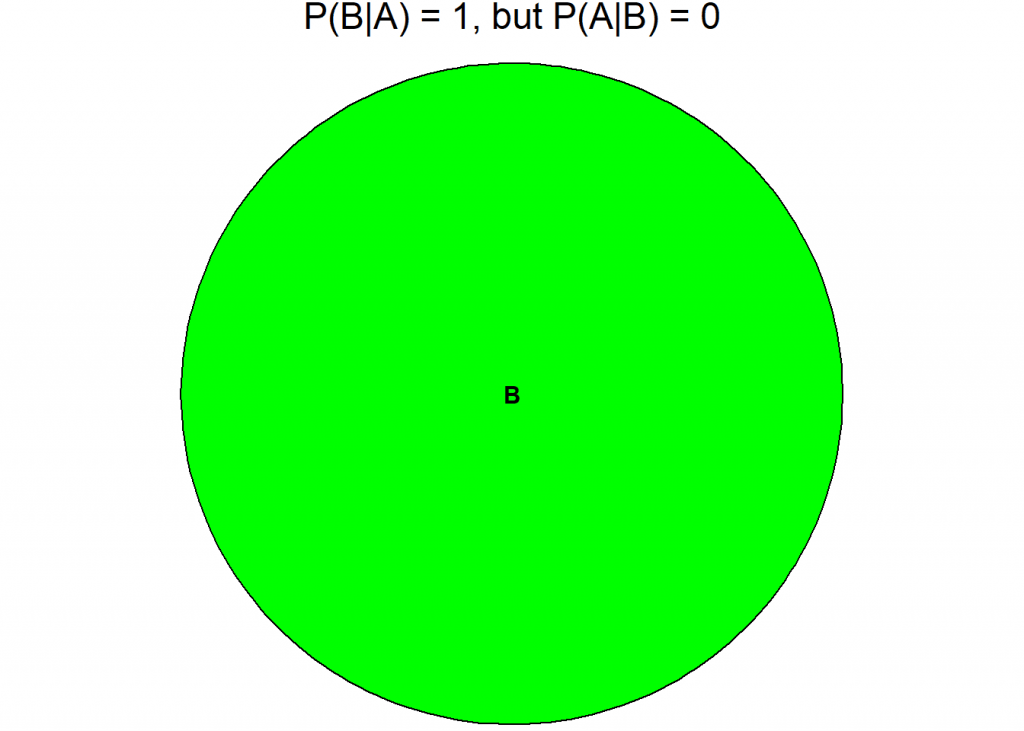Want to share your content on R-bloggers? click here if you have a blog, or here if you don't.In this post we are talking about one of the most unintuitive results of statistics: the so called false positive paradox which is an example of the so called base rate fallacy. It describes a situation where a positive test result of a very sensitive medical test shows that you have the respective disease… yet you are most probably healthy!

The reason for this is that the disease itself is so rare that even with a very sensitive test the result is most probably false positive: it shows that you have the disease yet this result is false, you are healthy.

The key to understanding this result is to understand the difference between two conditional probabilities: the probability that you have a positive test result when you are sick and the probability that you are sick in case you got a positive test result – you are interested in the last (am I really sick?) but you only know the first.

Now for some notation (the vertical dash means “under the condition that”, P stands for probability):

•: if you are sick () you will probably have a positive test result () – this is what we know
•: if you have a positive test result () you are probably not sick () – this is what we want to know

To calculate one conditional probability from the other we use the famous Bayes’ theorem:In the following example we assume a disease with an infection rate of 1 in 1000 and a test to detect this disease with a sensitivity of 99%. Have a look at the following code which illustrates the situation with Euler diagrams, first the big picture, then a zoomed-in version:

library(eulerr)

A <- 0.001 # prevalence of disease
BlA <- 0.99 # sensitivity of test

B <- A * BlA + (1 - A) * (1 - BlA) # positive test (specificity same as sensitivity)
AnB <- BlA * A
AlB <- BlA * A / B # Bayes's theorem
#AnB / B # Bayes's theorem in different form

C <- 1 # the whole population
main <- paste0("P(B|A) = ", round(BlA, 2), ", but P(A|B) = ", round(AlB, 2))

set.seed(123)
fit1 <- euler(c("A" = A, "B" = B, "C" = C, "A&B" = AnB, "A&C" = A, "B&C" = B, "A&B&C" = AnB), input = "union")
plot(fit1, main = main, fill = c("red", "green", "gray90"))fit2 <- euler(c("A" = A, "B" = B, "A&B" = AnB), input = "union")
plot(fit2, main = main, fill = c("red", "green"))As you can see although this test is very sensitive when you get a positive test result the probability of you being infected is only 9%!

In the diagrams C is the whole population and A are the infected individuals. B shows the people with a positive test result and you can see in the second diagram that almost all of the infected A are also part of B (the brown area = true positive), but still most ob B are outside of A (the green area), so although they are not infected they have a positive test result! They are false positive.

The red area shows the people that are infected (A) but get a negative test result, stating that they are healthy. This is called false negative. The grey area shows the people who are healthy and get a negative test result, they are true negative.Due to the occasion we are now coming to an even more extreme example: did Jesus rise from the dead? It is inspired by the very good essay “A drop in the sea”: Don’t believe in miracles until you’ve done the math.

Let us assume that we had very, very reliable witnesses (as a side note what is strange though is that the gospels cannot even agree on how many men or angels appeared at the tomb: it is one angel in Matthew, a young man in Mark, two men in Luke and two angels in John… but anyway), yet the big problem is that not many people so far have been able to defy death. I have only heard of two cases: supposedly the King of Kings (Jesus) but also of course the King himself (Elvis!), whereby sightings of Elvis after his death are much more numerous than of Jesus (just saying…)

Have a look at the following code (source for the number of people who have ever lived: WolframAlpha)

A <- 2/108500000000 # probability of coming back from the dead (The King = Elvis and the King of Kings = Jesus)
BlA <- 0.9999999 # sensitivity of test -> very, very reliable witnesses (many more in case of Elvis

B <- A * BlA + (1 - A) * (1 - BlA) # positive test = witnesses say He rose
AnB <- BlA * A
AlB <- BlA * A / B # Bayes's theorem

C <- 1 # all people
main <- paste0("P(B|A) = ", round(BlA, 2), ", but P(A|B) = ", round(AlB, 2))

fit1 <- euler(c("A" = A, "B" = B, "C" = C, "A&B" = AnB, "A&C" = A, "B&C" = B, "A&B&C" = AnB), input = "union")
plot(fit1, main = main, fill = c("red", "green", "gray90"))fit2 <- euler(c("A" = A, "B" = B, "A&B" = AnB), input = "union")
plot(fit2, main = main, fill = c("red", "green"))So, in this case C is the unfortunate group of people who have to go for good… it is us.As you can see although the witnesses are super reliable when they claim that somebody rose it is almost certain that they are wrong:

•: if Jesus rose () the very, very reliable witnesses would with a very high probability say so ()
•: if the very, very reliable witnesses said that Jesus rose () Jesus would still almost surely have stayed dead

Or in the words of the above mentioned essay:

No one is justified in believing in Jesus’s resurrection. The numbers simply don’t justify the conclusion.

But this chimes well with a famous Christian saying “I believe because it is absurd” (or in Latin “Credo quia absurdum”) – you can find out more about that in another highly interesting essay: ‘I believe because it is absurd’: Christianity’s first meme

Unfortunately this devastating conclusion is also true in the case of Elvis…